Best daily deals

# How to add cells in Excel

It's time to brush up on some basic math.
By
March 14, 2023

The most essential features in Excel are often the simplest ones. You’ll find that almost every project requires some degree of math. In this case, we’re talking about the essential skill of adding cells. However, there are quite a few different ways to accomplish your goal. Here are two quick ways to add cells in Excel.

To add cells in Excel, use the SUM() function. Enter the cells you want to add up inside the brackets or select a range of cells. Press Enter to see your results.

## How to add cells in Excel

The most basic way to add cells in Excel is to write a formula.

Start with an equals sign (=) followed by your cells separated by a plus sign (+). In our example, this reads as =A2+A3+A4.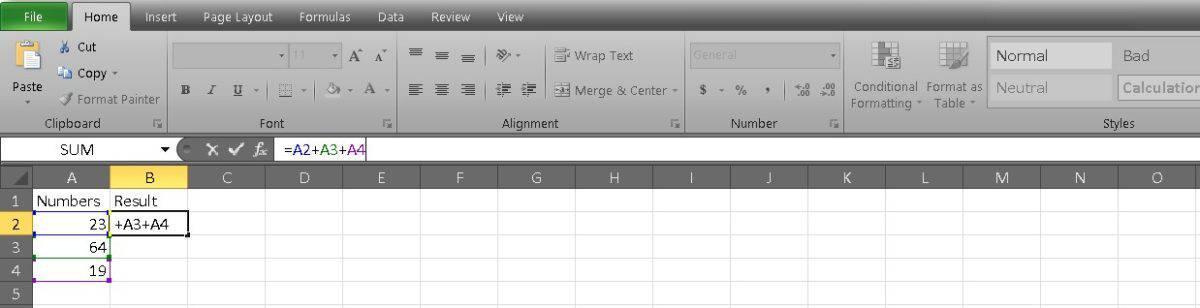Press Enter to get your result.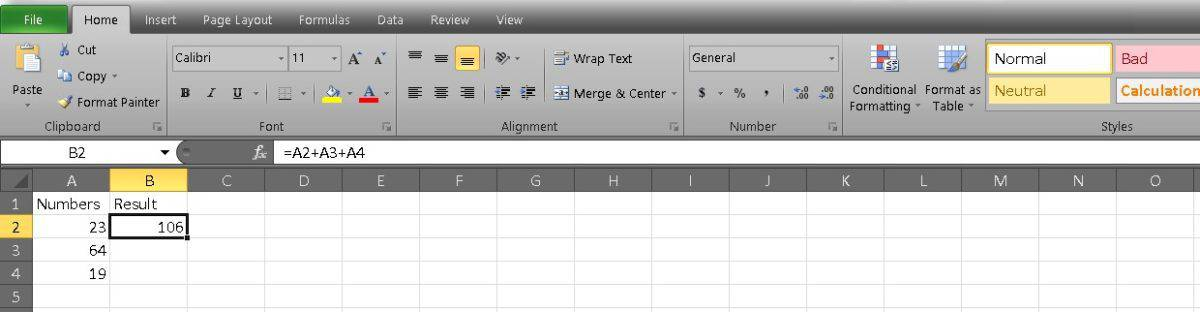This method is easiest if you add just a few cells and don’t mind typing them. However, you’ll probably find that using the SUM function to add long strings of cells is faster.

Start with the equals sign again, as this is how all formulas start. Then type SUM( to create your function.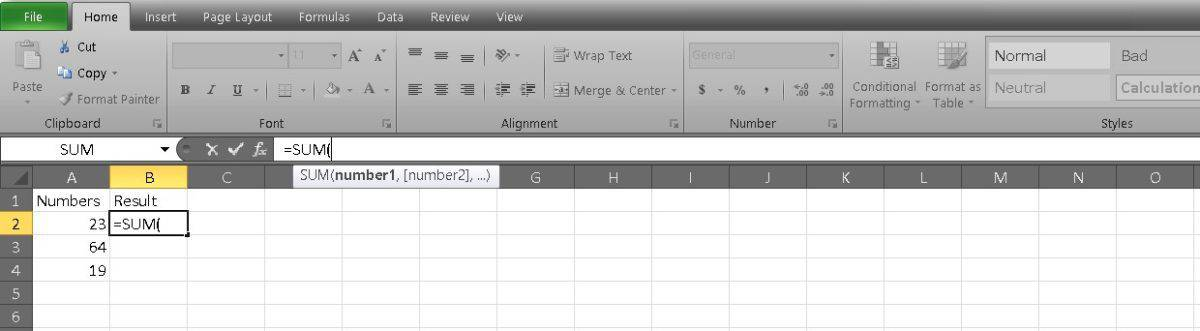Now you can type in the cells you want to add and divide them with commas. This would read as such: =SUM(A2,A3,A4).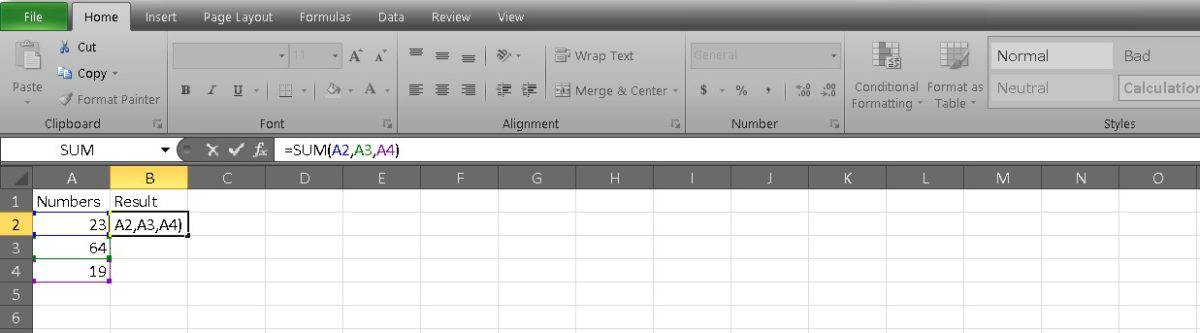If you’re adding a string of unbroken cells, you can separate them in your equation with a colon. This is easier if you have a long list because you can enter just the first and last cells. Our example looks like this: =SUM(A2:A4).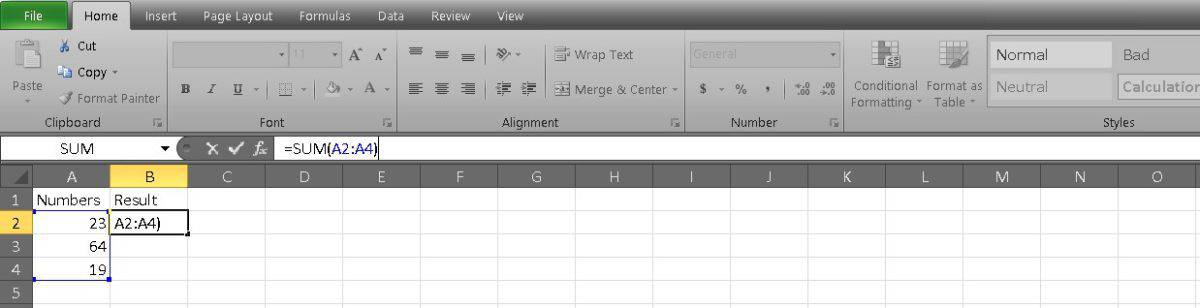A third way you can populate your formula is by clicking and dragging. After you’ve written =SUM, you can select the first cell you want to add and drag it to the last cell. Excel will do the rest and separate it with a colon.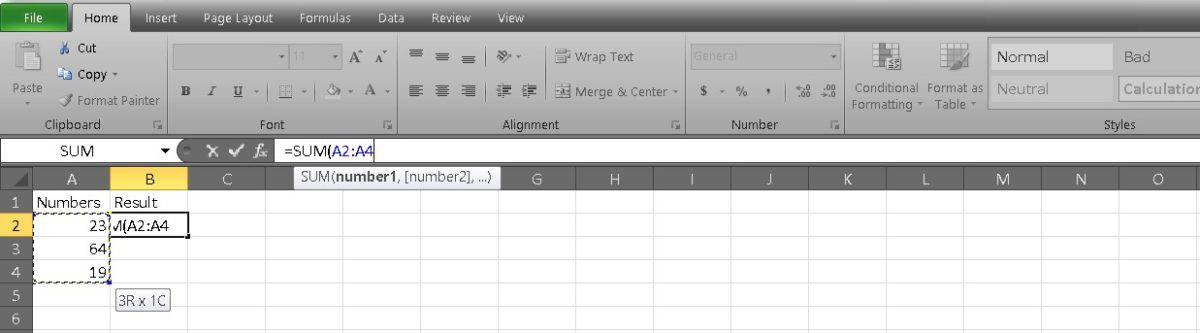You can even add up all of the cells in a column at once. In your formula, note the cells as A:A. Your formula bar will display as =SUM(A:A).

Press Enter after you’ve decided on your cells to see the result.There you have the quickest ways to add cells in Excel. If you want to learn how to sum and subtract in Google Sheets, we also have a guide fo that. Happy adding!

### FAQs

To add or subtract multiple cells, enter the plus (+) or minus (-) signs in between the cell numbers you want to combine into the function bar, and press Enter to display the results.

Use the formula =SUM(), with the cell numbers you want to add inside the brackets.

To add up cells in Excel, you can use the SUM function. Here’s how:

1. Select the cell where you want the result to appear.
2. Type “=SUM(” (without the quotes).
3. Select the range of cells you want to add up. You can do this by clicking and dragging your mouse over the cells or by manually typing in the range (e.g. A1:A10).
4. Type “)” (without the quotes) and press Enter.

The result should appear in the cell you selected in step 1. If you want to add up multiple ranges of cells, you can separate them with commas inside the SUM function. For example, “=SUM(A1:A10, C1:C10)” would add up the values in cells A1 through A10 and C1 through C10.